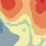# How to calculate spatial variability/semivariogram for each grid cell?

637
4
04-17-2019 07:46 AMby
New Contributor

Hello,

I have a surface of air pollutant levels and I would like to calculate the spatial variability/semivariogram for each grid cell. Does anyone know how to do it in Arcgis?

Thank you very much!

Liu

4 RepliesbyEsri Regular Contributor

Hi Liu,

The Moving Window Kriging geoprocessing tool is designed to calculate local semivariograms at particular locations.  The workflow you need to follow is:

1. If your grid is a raster, you will need to convert it to a point feature class using Raster To Point.
2. Use the Geostatistical Wizard to interpolate these points using kriging.  Choose the kriging type (Simple, ordinary, etc), define any options like detrending or transformation, and choose a semivariogram model (Exponential, Stable, K-Bessel, etc).  Do not worry about parameters like the range or sill yet; this interpolation is only to make a template for use in the next step.
3. Use Moving Window Kriging and provide the geostatistical layer created in step 2 as the model source.  You'll need to provide the locations where you want to calculate the semivariogram (use the same points you used to interpolate), and choose how many neighbors you want to use for each local calculation.

The output will be a point feature class containing (among other things), the nugget, range, and partial sill calculated at each location.  These are based on the kriging type and semivariogram model chosen in the Geostatistical Wizard and on the number of neighbors defined in Moving Window Kriging.

Let me know if you have any other questions or need any clarifications.

-Ericby
New Contributor

Hi Eric,

Thank you very much for your quick and detailed reply!!

I've tried your method and it worked very well. I have 2 further questions:

(1) for the "Maximum neighbors to include" when doing the moving window kriging. What does the neighbor mean? Does it mean the number of points (like neighbors of 10 = searching for 10 points around) or the unit of the map (like neighbors of 10=10 meters if the map is in a UTM projection)?

(2) the output attribute table has prediction, strErr, Nugget, Range, PartSill.  Which one is usually used to quantify the spatial variance, Nugget or Nugget+PartSill?

Thank you very much!byEsri Regular Contributor

(1) In Moving Window Kriging, the number of neighbors is the number of points that will be used to estimate the semivariogram parameters at each location.  To estimate the semivariogram parameters as a location, the software must use data from around the location (these points are called neighbors).  You want a sufficient number of neighbors to be able to estimate the semivariogram parameters accurately, but you also want the estimate to be local, so you don't want to use more neighbors than is necessary.  Using 30 points is usually sufficient.

(2) The partial sill plus the nugget will equal the variance of the data.  I'm not entirely clear what you mean by "spatial variance."  The semivariogram itself is what defines spatial covariance (ie, how correlated points are, given how far apart they are).by
New Contributor

That's exactly what I'm looking for. Thank you very much for your great help!!